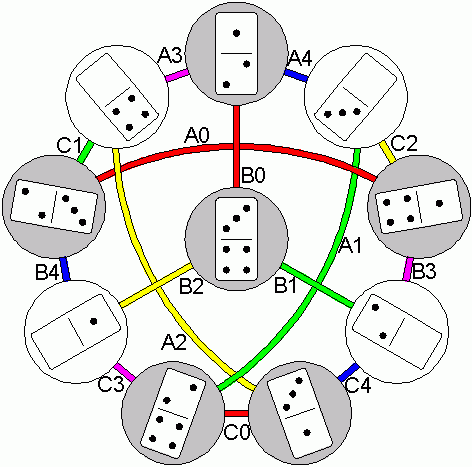Math Games Domino Graphs Ed Pegg Jr., December 15, 2003

Quite often, math is most effectively demonstrated via a game. Unfortunately, a game can often be eschewed for not being serious.

Take the following set of ten dominoes: 1-3, 2-4, 0-1, 2-3, 0-4, 1-2, 0-3, 1-4, 0-2, and 3-4. If we ignore the 3-4 for a moment, a ring of 9 dominoes can be made, where no two adjacent tiles have a number in common. See the outside ring in the below figure, in which all ten dominoes are shown as circular nodes. A colored edges indicates a legal connections. For example, dominoes 2-4 and 1-3 are connected by the red edge C0. A0, B0, and C0 are all red edges between domino nodes that lack a zero. A1, B1, and C1 are all green edges between domino nodes that lack a 1. There are three edges of each color, each representing one of the five numbers.Figure 1. The Petersen Graph, as a domino problem.

Is the ring of 10 dominoes possible, in which no two adjacent dominoes share a number? Suppose, for a moment, that it is possible. Ponder the gray and white nodes. Between usages of the red edges, the colors alternate grey-white-grey. Thus, between red edges, sequences with an even number of edges occur. If all three red edges get used, then the total cycle length is the sum of three even numbers, plus 3. That's an odd number, but 10 is even. We cannot use all three red edges in an even-length cycle. Similarly, none of the other colors can be used three times, in our supposed 10 domino ring. Every color needs to occur exactly twice.

Suppose that C0 isn't used. C3 and A1 would be necessary, along with A2 and C4. One of B1 or B2 must be used. If B2 is chosen, B4 cannot be used, forcing the usage of A4 since every color must be used twice. That gives the closed cycle C3-B2-B0-A4-A1, and we're stuck. If B1 is chosen, B3 cannot be used, forcing the usage of A3. That gives closed cycle C4-B1-B0-A3-A2, and we're stuck. It would appear that C0 is necessary, but the same sort of problem appears if A0 or B0 is chosen as the missing red edge. After eliminating all possibilities, we must conclude that no ring of ten dominoes exists. In alternate terminology, we conclude that the Petersen Graph is non-Hamiltonian.Figure 2. Various ways to draw a Petersen Graph.

A different problem involves planting 10 trees in 10 lines of 3 trees each, with each tree used in exactly three lines. If the trees are turned into ten dominoes, another famous graph can be made. Consider one of the lines, for example 1-2, 2-3, 1-3. The line itself can be identified by the numbers not on it, 0-4 in this case. Each line can be considered as an anti-domino. In the accompanying graph, red and blue indicate points and lines. A gray edge connects a point and a line, and vice-versa. Or a domino and an anti-domino.Figure 3. The Desargues 103 configuration, and the Desargues graph.

For a bigger graph, consider the Stomachion of Archimedes, which Bill Cutler recently solved (my 11-17-03 column). Most of the solutions split the pieces into two dominoes. Many times, a solution will have a subset of pieces in a symmetrical shape that can be flipped over or rotated. As a graph, we can connect any two solutions that related by one of these flips or rotations. Fan Chung and Ron Graham recently constructed that very graph. (I urge a visit to their page). Amazingly, all but two of the solutions are connected within this graph! More amazingly, Dr. Reviel Netz, the Palimpsest's investigator, has made a convincing argument that Archimedes did the exact same thing, 2200 years ago, based upon Archimedes notes for the problem.

If true, Archimedes was the first serious combinatorialist. You can see more in a column Gina Kolata wrote for the New York Times about the discoveries of Netz, Cutler, Chung, and Graham. Pity poor Archimedes. He tried to introduce a major new field of mathematics via a game, and was ignored and misunderstood for 2200 years. For math academia, that's gotta be a record.Figure 4. Two arrangements of order 6 domino graph.

References:

Chung, F. and Graham, R. "A tour of Archimedes' Stomachion". http://math.ucsd.edu/~fan/stomach/.

Coxeter, H. S. M. The Beauty of Geometry. Dover Publications, Inc., 1968. p 108-148.

Friedman, E. Tree Planting Problems. http://www.stetson.edu/~efriedma/trees/.

Holton D.A., Sheehan J. The Petersen Graph. Cambridge University Press, 1992.

Kolata, G. "In Archimedes' Puzzle, a New Eureka Moment", New York Times, December 14, 2003. http://www.nytimes.com/2003/12/14/science/14MATH.html.

Pegg, E. "The Loculus of Archimedes, Solved", Math Games, November 17, 2003. http://www.maa.org/editorial/mathgames/mathgames_11_17_03.html.

Weisstein, E W. Configuration, Desargues Graph, Petersen Graph. Eric Weisstein's World of Mathematics. http://mathworld.wolfram.com/.

West, D. Introduction to Graph Theory, 2nd Edition. Prentice Hall, 2001. p 13.

Wolfram, S. A New Kind of Science. Champaign, IL: Wolfram Media, 2002. p 1032.

Mathematica Code: